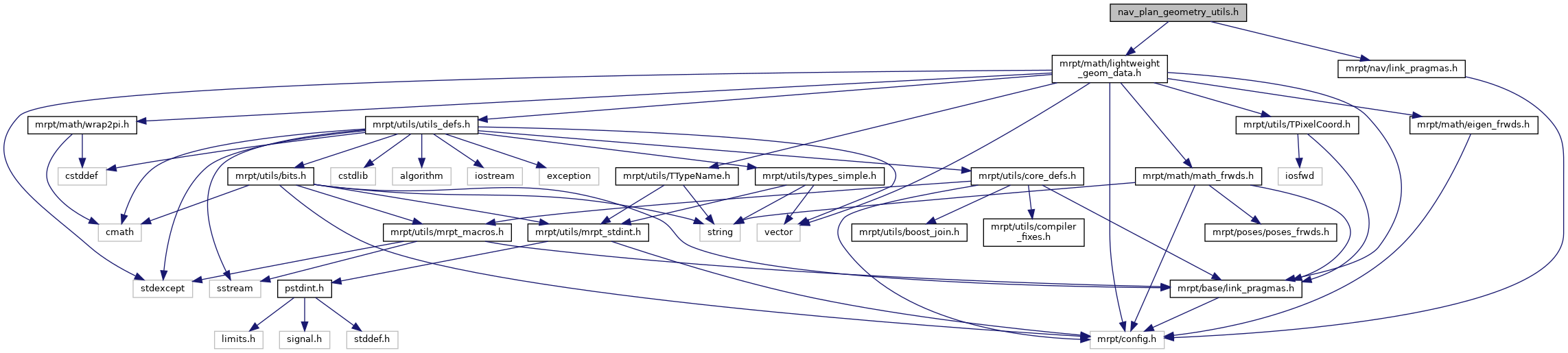Main MRPT website > C++ reference for MRPT 1.5.6
nav_plan_geometry_utils.h File Reference
`#include <mrpt/math/lightweight_geom_data.h>`
`#include <mrpt/nav/link_pragmas.h>`
Include dependency graph for nav_plan_geometry_utils.h:This graph shows which files directly or indirectly include this file:Go to the source code of this file.

Namespaces

mrpt
This is the global namespace for all Mobile Robot Programming Toolkit (MRPT) libraries.

mrpt::nav

Functions

bool NAV_IMPEXP mrpt::nav::collision_free_dist_segment_circ_robot (const mrpt::math::TPoint2D &p_start, const mrpt::math::TPoint2D &p_end, const double robot_radius, const mrpt::math::TPoint2D &obstacle, double &out_col_dist)
Computes the collision-free distance for a linear segment path between two points, for a circular robot, and a point obstacle (ox,oy). More...

bool NAV_IMPEXP mrpt::nav::collision_free_dist_arc_circ_robot (const double arc_radius, const double robot_radius, const mrpt::math::TPoint2D &obstacle, double &out_col_dist)
Computes the collision-free distance for a forward path (+X) circular arc path segment from pose (0,0,0) and radius of curvature R (>0 -> +Y, <0 -> -Y), a circular robot and a point obstacle (ox,oy). More...

 Page generated by Doxygen 1.8.6 for MRPT 1.5.6 Git: 4c65e84 Tue Apr 24 08:18:17 2018 +0200 at mar abr 24 08:26:17 CEST 2018